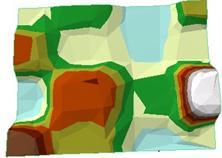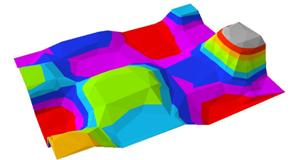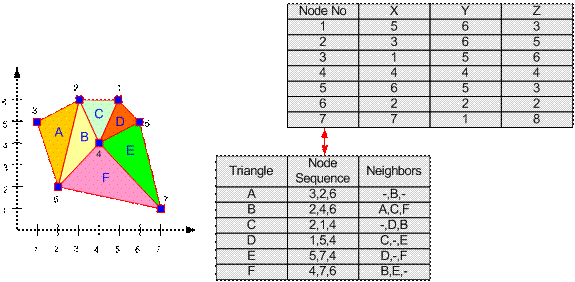## Triangulated Irregular Network ModelTIN or Triangulated Irregular Network stored GIS data for 3D surface model. The basic unit is a triangle. Because a triangle consists of three lines connecting three nodes, each triangle will have three neighbors (except those on the side or periphery). The triangle is represented by a sequence of three nodes. Each triangle may have other associated attributes such as population density, crime rate, etc. in another table.Preferable reference for this tutorial is

Teknomo, Kardi (2015) Introduction to GIS. https:\\people.revoledu.com\kardi\ tutorial\GIS\